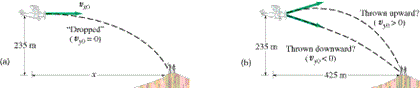# Problem: A rescue plane wants to drop supplies to isolated mountain climbers on a rocky ridge 235 m below. The plane is traveling horizontally with a speed of 265 km/h (73.6 m/s).(a) How far in advance of the recipients (horizontal distance) must the goods be dropped (Fig. 3-41 a)?(b) Suppose, instead, that the plane releases the supplies a horizontal distance of 425 m in advance of the mountain climbers. What vertical velocity (up or down) should the supplies be given so that they arrive precisely at the climbers' position (Fig. 3-41 b)? (Horizontal velocity is the same as in part (a)).Magnitude: ___ m/sDirection:a. upb. down(c) With what speed (magnitude of impact velocity) do the supplies land in the latter case?m/s

###### FREE Expert Solution

UAM equations:

Horizontal distance:

Vector magnitude:

$\overline{)\mathbf{|}\stackrel{\mathbf{⇀}}{\mathbf{v}}\mathbf{|}{\mathbf{=}}\sqrt{{{\mathbf{v}}_{\mathbf{x}}}^{\mathbf{2}}\mathbf{+}{{\mathbf{v}}_{\mathbf{y}}}^{\mathbf{2}}}}$

(a)

The time is unknown:

• vx  =73.6 m/s
• Δy = - 235 m
• g = 9.8 m/s2
• v0,y = 0 m/s
• vf,y = ?
• Δt = ?
• Δx = ?

Solving for time:

Δy = v0,yt - (1/2)gt2

-235 = 0 - (1/2) × 9.8 × t2

97% (233 ratings)###### Problem DetailsA rescue plane wants to drop supplies to isolated mountain climbers on a rocky ridge 235 m below. The plane is traveling horizontally with a speed of 265 km/h (73.6 m/s).

(a) How far in advance of the recipients (horizontal distance) must the goods be dropped (Fig. 3-41 a)?

(b) Suppose, instead, that the plane releases the supplies a horizontal distance of 425 m in advance of the mountain climbers. What vertical velocity (up or down) should the supplies be given so that they arrive precisely at the climbers' position (Fig. 3-41 b)? (Horizontal velocity is the same as in part (a)).

Magnitude: ___ m/s

Direction:

a. up

b. down

(c) With what speed (magnitude of impact velocity) do the supplies land in the latter case?

m/s